## 磁致伸缩主被动隔振装置中的磁机耦合效应研究 1)

* 上海交通大学机械系统与振动国家重点实验室,上海 200240

† 哈尔滨工业大学深圳校区理学院,深圳 518055

## RESEARCH ON THE MAGNETO-MECHANICAL EFFECT IN ACTIVE AND PASSIVE MAGNETOSTRICTIVE VIBRATION ISOLATOR 1)

Niu Muqing*,†, Yang Bintang*, Yang Yikun*, Meng Guang*, Chen Liqun*,2)

* State Key Laboratory of Mechanical System and Vibration, Shanghai Jiao Tong University, Shanghai 200240, China

† School of Science, Harbin Institute of Technology in Shenzhen, Shenzhen 518055, China

 基金资助: 国家自然科学基金资助项目.  51775349

Received: 2018-08-2   Accepted: 2018-11-6   Online: 2019-03-18

2)陈立群,教授,主要研究方向：非线性动力学与振动控制.E-mail：chenliqun@hit.edu.cnAbstract

The actuation system, which is composed of magnetostrictive actuator and compliant displacement amplifier, has the advantages of high precision and large actuation force. It is connected in parallel with passive vibration isolator. The resulting active and passive vibration isolator can make up for the deficiencies of passive isolator on low-frequency and micro-amplitude conditions. In this paper, a nonlinear magnetostrictive actuation model is proposed based on Jiles-Atherton model. Magneto-mechanical effect is comprehensively characterized by being decomposed into stress related effects on effective field, magnetization, magnetostriction and Young's modulus. A dynamic model of the isolator is established considering the coupling effects between active isolator and passive isolator. With the coupling effect, the performance of actuation system is related to passive isolator parameters. With higher passive isolator stiffness, the actuation displacement decreases and the required actuation force increases. The coupling effect also leads to the change of equivalent stiffness of the isolator due to the ∆E effect of magnetostrictive material. The influence of coupling effects can be weakened by parameter design of compliant amplifier. The performances of the active and passive vibration isolator are validated by numerical simulation. Three kinds of vibration frequencies are used, which are below, around and beyond natural frequency of the isolator, respectively. Compared to passive vibration isolator, better vibration isolation performances are acquired by adding active vibration isolator on all three conditions. And the calculation results show that the proposed model considering magneto-mechanical effect can reach a higher accuracy.

Keywords： vibration isolation ; magnetostrictive material ; magneto-mechanical effect ; ∆E effect

Niu Muqing, Yang Bintang, Yang Yikun, Meng Guang, Chen Liqun. RESEARCH ON THE MAGNETO-MECHANICAL EFFECT IN ACTIVE AND PASSIVE MAGNETOSTRICTIVE VIBRATION ISOLATOR 1). Chinese Journal of Theoretical and Applied Mechanics[J], 2019, 51(2): 324-332 DOI:10.6052/0459-1879-18-254

## 1 磁致伸缩主被动隔振装置建模

### 图1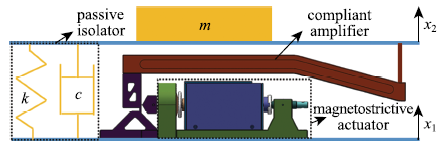Fig.1   Structure of magnetostrictive isolator

### 图2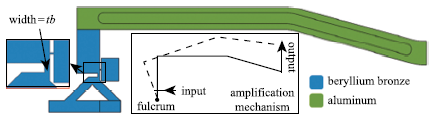Fig.2   Structure of compliant amplifier

### 1.2 磁致伸缩驱动建模

$$H_{\rm e} = H + \alpha M$$

$$M_{\rm an} = M_{\rm s} \left[ {\coth \left( {\frac{H_{\rm e} }{a}} \right)-\frac{a}{H_{\rm e} }} \right]$$

$$\frac{{\rm d}M}{{\rm d}H_{\rm e} } = r\frac{{\rm d}M_{\rm an} }{{\rm d}H_{\rm e} } + \frac{M_{\rm an}-M}{w\delta }$$

$$\lambda = \frac{3\lambda _{\rm s} M^2}{2M_{\rm s}^2 }$$

$$\varepsilon = \frac{\sigma-\sigma _0 }{E_{\rm m} } + \lambda$$

$M = \left( {1-r} \right)M_{\rm irr} + rM_{\rm an}$

$\frac{{\rm d}M_{\rm irr} }{{\rm d}H_{\rm e} } = \frac{1}{1-r}\frac{M_{\rm an}-M}{w}$

$H_{\rm e} = H + \alpha M + \frac{3}{2}\frac{\sigma }{\mu _0 }\frac{\partial \lambda }{\partial M}$

$\frac{{\rm d}M_{\rm irr} }{{\rm d}\sigma } = \frac{\sigma }{E\xi }\left( {M_{\rm an}-M_{\rm irr} } \right)$

$\frac{{d}M}{{\rm d}t} = r\frac{{\rm d}M_{\rm an} }{{\rm d}t} + \left( {1-r} \right)\cdot\\ \left( \frac{ \partial M_{\rm irr} }{ \partial H_{\rm e} }\frac{{\rm d}H_{\rm e} }{{\rm d}t} + \frac{ \partial M_{\rm irr} }{ \partial \sigma }\frac{{\rm d}\sigma }{{\rm d}t} \right)$

$\frac{{\rm d}M}{{\rm d}t} = \frac{M_{\rm an}-M}{w}\frac{{\rm d}H_{\rm e} }{{\rm d}t} +\\ \frac{\sigma }{E\xi }\left( {M_{\rm an}-M} \right)\frac{{\rm d}\sigma }{{\rm d}t} + r\frac{{\rm d}M_{\rm an} }{{\rm d}t}$

$\lambda = [1-\frac{1}{2} tanh ( \frac{2 \sigma}{\sigma _{s}}) ] \frac {\lambda _{s} M^2}{M_{s}^2 }$

$\epsilon = \frac{\sigma-\sigma _0 }{E_{\rm s} } + \frac{\lambda _{\rm s} }{2}\tanh \left( {\frac{2\sigma }{\sigma _{\rm s} }} \right)-\frac{\lambda _{\rm s} }{2}\tanh \left( {\frac{2\sigma _0 }{\sigma _{\rm s} }} \right) + \lambda \quad$

$H_{\rm e} = H + \alpha M + \left[ {2-\tanh \left( {\frac{2\sigma }{\sigma _{\rm s} }} \right)} \right]\frac{3\sigma \lambda _{\rm s} M}{2\mu _0 M_{\rm s}^2 }$

$\frac{1}{E} = \frac{1}{E_{\rm s} } + \frac{\lambda _{\rm s} }{\sigma _{\rm s} }\left( {1-\frac{M^2}{M_{\rm s}^2 }} \right)\left[ {1-\tanh ^2\left( {\frac{2\sigma }{\sigma _{\rm s} }} \right)} \right]$

### 1.3 隔振装置建模

$x_{\rm a}= L_{\rm T} \varepsilon$

$F_{\rm a} =-A_{\rm T} \sigma$

$\left[ {{\begin{array}{*{20}c} {p_{11} } & {p_{12} } \\ {p_{21} } & {p_{22} } \\ \end{array} }} \right]\left[ {{\begin{array}{*{20}c} {x_{\rm a} } \\ {x_2-x_1 } \\ \end{array} }} \right] = \left[ {{\begin{array}{*{20}c} {F_{\rm a} } \\ {-F} \\ \end{array} }} \right]$

$F-k\left( {x_2-x_1 } \right)-c\left( {\frac{{\rm d}x_2 }{{\rm d}t}-\frac{{\rm d}x_1 }{{\rm d}t}} \right)-m\frac{{\rm d}^2x_2 }{{\rm d}t^2} = 0$

$\left. {{\begin{array}{l} {H_{\rm e} = H + \alpha M + \left[ {2-\tanh \left( {\frac{2\sigma }{\sigma _{\rm s} }} \right)} \right]\frac{3\sigma \lambda _{\rm s} M}{2\mu _0 M_{\rm s}^2 }} \\[5mm] {M_{\rm an} = M_{\rm s} \left[ {\coth \left( {\frac{H_{\rm e} }{a}} \right)-\frac{a}{H_{\rm e} }} \right]} \\[5mm] \frac{{\rm d}M}{{\rm d}t} = \frac{M_{\rm an}-M}{w}\frac{{\rm d}H_{\rm e} }{{\rm d}t} +\\[3mm] \qquad \frac{\sigma }{E\xi }\left( {M_{\rm an}-M} \right)\frac{{\rm d}\sigma }{{\rm d}t} + r\frac{{\rm d}M_{\rm an} }{{\rm d}t} \\[5mm] {\frac{1}{E} = \frac{1}{E_{\rm s} } + \frac{\lambda _{\rm s} }{\sigma _{\rm s} }\left( {1-\frac{M^2}{M_{\rm s}^2 }} \right)\left[ {1-\ tanh ^2\left( {\frac{2\sigma }{\sigma _{\rm s} }} \right)} \right]} \\[5mm] {\lambda = \left[ {1-\frac{1}{2}\tanh \left( {\frac{2\sigma }{\sigma _{\rm s} }} \right)} \right]\frac{\lambda _{\rm s} M^2}{M_{\rm s}^2 }} \\[5mm] \frac{x_{\rm a} }{L_{\rm T} } = \frac{\sigma-\sigma _0 }{E_{\rm s} } + \frac{\lambda _{\rm s} }{2}\tanh \left( {\frac{2\sigma }{\sigma _{\rm s} }} \right)-\\[3mm] \qquad \frac{\lambda _{\rm s} }{2}\tanh \left( {\frac{2\sigma _0 }{\sigma _{\rm s} }} \right) + \lambda \\[5mm] {\left[ {{\begin{array}{*{20}c} {p_{11} } & {p_{12} } \\ {p_{21} } & {p_{22} } \\ \end{array} }} \right]\left[ {{\begin{array}{*{20}c} {x_{\rm a} } \\ {x_2-x_1 } \\ \end{array} }} \right] = \left[ {{\begin{array}{*{20}c} {-A_{\rm T} \sigma } \\ {-F} \\ \end{array} }} \right]} \\[5mm] {F-k\left( {x_2-x_1 } \right)-c\left( {\frac{{\rm d}x_2 }{{\rm d}t}-\frac{{\rm d}x_1 }{{\rm d}t}} \right)-m\frac{{\rm d}^2x_2 }{{\rm d}t^2} = 0} \\ \end{array} }} \right\}$

## 2 主、被动隔振装置间耦合作用分析

### 2.1 被动隔振参数对驱动性能的影响

$F-kx_2 = 0$

$\frac{F_{\rm a} }{x_{\rm a} } = \frac{-\sigma A_{\rm T} }{\varepsilon L_{\rm T} } = p_{11}-\frac{p_{12} p_{21} }{p_{22} + k}$

$\frac{-\sigma A_{\rm T} }{L_{\rm T} \left( {p_{11}-\frac{p_{12} p_{21} }{p_{22} + k}} \right)} = \frac{\sigma-\sigma _0 }{E_{\rm s} } + \frac{\lambda _{\rm s} }{2}\tanh \left( {\frac{2\sigma }{\sigma _{\rm s} }} \right)-\\ \frac{\lambda _{\rm s} }{2}\tanh \left( {\frac{2\sigma _0 }{\sigma _{\rm s} }} \right) + \left[ {1-\frac{1}{2}\tanh \left( {\frac{2\sigma }{\sigma _{\rm s} }} \right)} \right]\frac{\lambda _{\rm s} M^2}{M_{\rm s}^2 }$

### 图3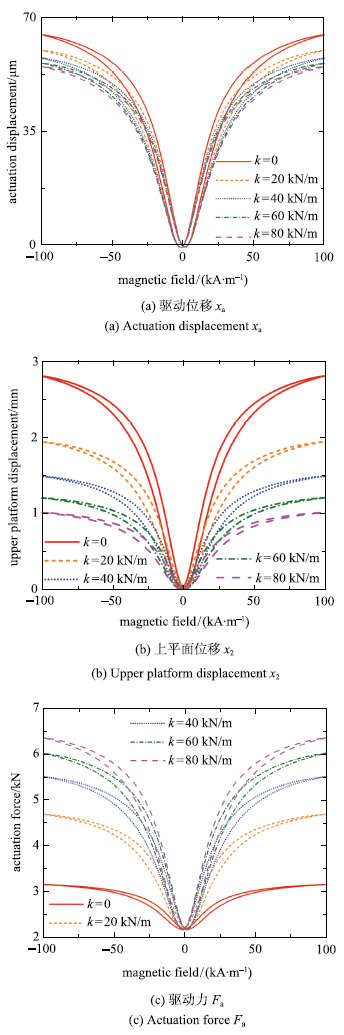Fig.3   Actuator output with different k value

k值越大,驱动位移越小,如图3(a)所示.不同k值下柔顺机构的位移放大倍数也不同,具体关系参见文献,k值越大,放大倍数越小,因此上平面位移的差异相对于驱动位移更大,k=0时的位移约为k=80 kN/m时的2.74倍,如图3(b)所示.此外,k值越大,驱动器所需要提供的驱动力也越大,如图3(c)所示,驱动力要求过高容易导致磁致伸缩材料尺寸过大、柔顺机构内应力过大等问题.在高频工况下,除弹簧刚度外,阻尼和负载质量也会通过磁机耦合影响磁致伸缩驱动器输出.

Table 1  The relation between Fa/Xa and tb

tb/mmFa-Xa-1 /(N-m-1) (k=0)Fa.Xa-1/(N.m-1)
(k=80 kA/m)
P11 / (N-m-1)
2.81.6X1077.9X1071.3X108
56.3X1071.2X1082.0X108
101.7X1082.0X1082.4X108
152.0X1082.2X1082.6X108

### 2.2 变刚度效应对被动隔振性能的影响

$k_{\rm eq} = E\frac{A_{\rm T} }{L_{\rm T} }$

$$k_{\rm sys} = k + p_{22}-\frac{p_{12} p_{21} }{k_{\rm eq} + p_{11} }$$

$$f_{\rm na} = \sqrt {\frac{k_{\rm sys} }{m}}$$

### 图4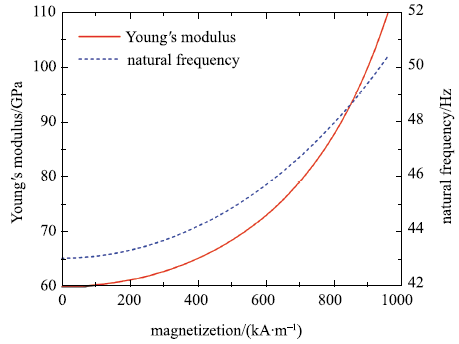Fig.4   $\Delta E$ effect and natural frequency change

Table 2  The relation between ksys and tb

tb/mm(k+P22)/ (N-m-1)ksys/(N-m-1)
(keq= 100 N/mm)
ksys/(N-m-1)
(keq = 300 N/mm)
2.86.9X1044.0X1045.4X104
24.4X1043.1X1043.8X104
1.52.8X1042.4X1042.7X104
11.7X1041.6X1041.6X104

## 3 主被动隔振数值仿真

### 图5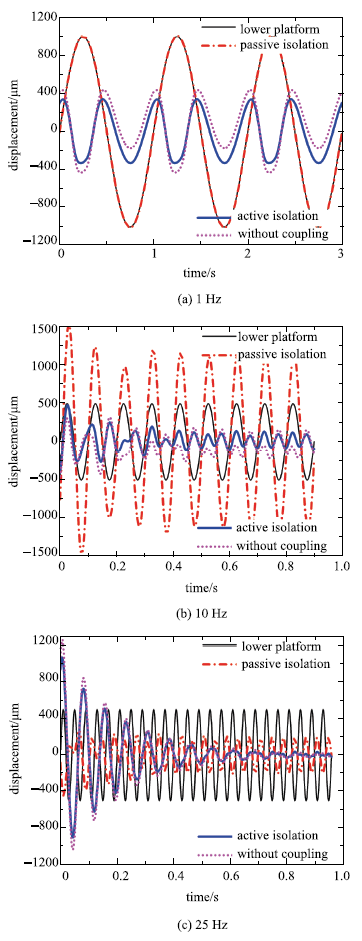Fig.5   Numerical results for vibration isolation

## 4 结 论

The authors have declared that no competing interests exist.

## 参考文献 原文顺序 文献年度倒序 文中引用次数倒序 被引期刊影响因子

URL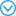( Cao Dengqing, Bai Kunchao, Ding Hu , et al.

Advances in dynamics and vibration control of large-scale flexible spacecraft

Chinese Journal of Theoretical and Applied Mechanics, 2019,51(1):1-13 (in Chinese))

URL( Dong Yaohai. Micro-Vibration of Spacecraft-Theory and Practice. Beijing: China Aerospace Press, 2015 (in Chinese))

URL( Lu Zeqi, Chen Liqun .

Some recent progresses in nonlinear passive isolations of vibrations

Chinese Journal of Theoretical and Applied Mechanics, 2017,49(3):550-564 (in Chinese))

URLURL( Li Shuai, Zhou Jilei, Ren Chuanbo , et al.

The research of time delay vibration control with time-varying parameters

Chinese Journal of Theoretical and Applied Mechanics, 2018,50(1):99-108 (in Chinese))

URLJoule J .

On a new class of magnetic forces

Ann Electr Magn Chem, 1842,8(1842):219-224

( Mao Jianqin. Dynamics and Control of Smart Structure. Beijing: Science Press, 2013 (in Chinese))

Huber JE, Fleck NA, Ashby MF .

The Selection of Mechanical Actuators Based on Performance Indices

Proceedings Mathematical Physical & Engineering Sciences, 1997,453(1965):2185-2205

URLA method is presented for selecting the type of actuator best suited to a given task, in the early stages of engineering design. The selection is based on matching performance characteristics of the actuator, such as force and displacement, to the requirements of the given task. The performance characteristics are estimated from manufacturers' data and from simple models of performance limitation such as heat generation and resonance. Characteristics are presented in a graphical form which allows for a direct and systematic comparison of widely different systems of actuation. The actuators considered include man-made actuators (such as hydraulic, solenoid and shape memory alloy) and naturally occurring actuators (such as the muscles of animals and plants).

A novel low-power linear magnetostrictive actuator with local three-phase excitation

IEEE/ASME Transactions on Mechatronics, 2010,15(2):299-307Development of a novel low-power linear magnetostrictive actuator is presented in this paper. The magnetostrictive material used here is Terfenol-D, which is an alloy of the formula TbDyFe. In response to a traveling magnetic field inside the Terfenol-D element, it moves in the opposite direction with a peristaltic motion. The proposed design offers the flexibility to operate the actuator in various configurations including local and conventional three-phase excitation. In this paper, we demonstrate that the power consumption can be reduced significantly by the local excitation approach. A new force-transmission assembly incorporates spring washers to avoid the wear due to the sudden collision of the Terfenol-D element with the force-transmission assembly. The closed-loop control system was implemented using relay control, which resulted in an optimal closed-loop performance. The magnetostrictive motor has demonstrated a 410-N load capacity with a travel range of 45 mm, and the present maximum speed is 9 mm/min. The low speed is due to the local three-phase operation mode, and it could be increased to 60 mm/min by using the conventional three-phase operation. The maximum power consumption by the motor is 95 W.

Xue G, Zhang P, Li X , et al.

A review of giant magnetostrictive injector (GMI)

Sensors & Actuators A Physical, 2018,273:159-181

URLGiant magnetostrictive material is a kind of excellent engineering material for its large magnetostriction, fast response speed and high energy density. And giant magnetostrictive injector which employs giant magnetostrictive material driving an electronic controlled injector may be one new promising injector. With more adjustable injection rate, giant magnetostrictive injector can promote energy utilization and reduce pollution emission. This paper provides a detailed overview of the research status of the giant magnetostrictive injector. Some important background information as the properties of giant magnetostrictive material, designing methods of giant magnetostrictive actuator and working circumstances of an electronic controlled injector are introduced firstly. Then the structure design, physical model, numerical simulation and power driver design for the giant magnetostrictive injector are reviewed especially. The summaries may support much useful information for the researchers of giant magnetostrictive injector. Furthermore, some general techniques like bias field utilizing skill, fast driving and simple modeling methods etc. are available to more research fields beyond the limitations of giant magnetostrictive injector.

Zhu Y, Yang X, Fu T .

Dynamic modeling and experimental investigations of a magnetostrictive nozzle-flapper servovalve pilot stage

Proceedings of the Institution of Mechanical Engineers Part I Journal of Systems & Control Engineering, 2016,230(3):197-207

Yoshioka H, Shinno H, Sawano H .

A newly developed rotary-linear motion platform with a giant magnetostrictive actuator

CIRP Annals-Manufacturing Technology, 2013,62(1):371-374

URLDemands for machining and measuring three-dimensional geometries have recently increased in a variety of industries. In order to meet such demands, it is necessary to develop a compact versatile high performance spindle system. This paper presents a newly developed rotary motion platform combined with a linear motion mechanism driven by a giant magnetostrictive actuator. The developed platform can be characterized by a compact structure, a noncontact structure, and high accuracy. Performance evaluation results confirm that the developed platform provides precise linear motion during rotating.

Park G, Bement MT, Hartman DA , et al.

The use of active materials for machining processes: A review

International Journal of Machine Tools & Manufacture, 2007,47(15):2189-2206

URLThis paper provides a review of active materials in the context of applications to manufacturing machining processes. The important concepts and background of active materials are briefly introduced. After which, the applications of these materials are discussed as applied to relevant themes in machining processes. A brief overview of research work on experimental and theoretical studies on various process monitoring and control is considered, and several research papers on these topics are cited. This paper concludes with a discussion of future research areas and a suggests path forward.

Sun X, Yang B, Zhao L , et al.

Optimal design and experimental analyses of a new micro-vibration control payload-platform

Journal of Sound & Vibration, 2016,374:43-60

URLThis paper presents a new payload-platform, for precision devices, which possesses the capability of isolating the complex space micro-vibration in low frequency range below 5Hz. The novel payload-platform equipped with smart material actuators is investigated and designed through optimization strategy based on the minimum energy loss rate, for the aim of achieving high drive efficiency and reducing the effect of the magnetic circuit nonlinearity. Then, the dynamic model of the driving element is established by using the Lagrange method and the performance of the designed payload-platform is further discussed through the combination of the controlled auto regressive moving average (CARMA) model with modified generalized prediction control (MGPC) algorithm. Finally, an experimental prototype is developed and tested. The experimental results demonstrate that the payload-platform has an impressive potential of micro-vibration isolation.

( Duan Bowen . Dynamic Analysis and Control of an Active Power-train Mount Based on Magnetostrictive Actuator. [Master Thesis]. Nanjing: Nanjing University of Aeronautics & Astronautics, 2016 (in Chinese))

Nakamura Y, Nakayama M, Masuda K , et al.

Development of active six-degrees-of-freedom microvibration control system using giant magnetostrictive actuators

Smart Materials & Structures, 2000,9(2):175-185

Nakamura Y, Nakayama M, Yasuda M , et al.

Development of active six-degrees-of-freedom micro-vibration control system using hybrid actuators comprising air actuators and giant magnetostrictive actuators

Smart Materials & Structures, 2006,15(4):1133-1142.

URLIn recent years, there has been an increasing demand for micro-vibration free space from industrial and scientific organizations. In response to this demand, several active micro-vibration control systems utilizing various types of actuators have been developed and applied in various environments and to a number of machines. The authors have developed an active micro-vibration control system with six degrees of freedom using giant magnetostrictive (GMS) actuators and actually have applied it to an FIB (focused ion beam).

Geng Z J, Haynes L S .

Six degree-of-freedom active vibration control using the Stewart platforms

IEEE Transactions on Control Systems Technology, 2002,2(1):45-53

Braghin F, Cinquemani S, Resta F .

A model of magnetostrictive actuators for active vibration control

Sensors & Actuators A Physical, 2011,165(2):342-350

URLOne of the most frequent application of magnetostrictive actuator technology is the active structural vibration control (AVC). Magnetostrictive actuators (MA) can deliver high-output forces and relatively high displacements (compared to other emerging actuator technologies) and can be driven at high frequencies: these characteristics make them suitable for a variety of vibration control applications. The use of this technology, however, requires an accurate knowledge of the dynamics of such actuators. The paper introduces a linear model of magnetostrictive actuators hold in a range of frequencies below 2 kHz useful in real time application as AVC. The hypotesis supporting the linearity of the systems are discussed and the theoretical model is presented. Finally the model is validated by testing two different models of magnetostrictive actuators and comparing experimental results with the theoretical ones.

Zhou HM, Zheng XJ, Zhou YH .

Active vibration control of nonlinear giant magnetostrictive actuators

Smart Materials & Structures, 2006,15(3):792-798

URLA nonlinear constitutive model-based vibration control system for giant magnetostrictive actuators (Terfenol-D) is presented in this paper. Such actuators utilize the realignment of magnetic moments in response to applied magnetic fields to generate strains in the material. It has been found that the strains and forces generated in this manner are significantly larger than those produced by many other smart materials, associated with significant and complex nonlinear relations among the quantities of applied magnetic field, strain, and compressive pre-stress. Based on the negative feedback control law and the analytical expressions of the nonlinear constitutive model of Terfenol-D rods, here, the effectiveness of real control systems for suppressing a vibration is confirmed by the simulation results on a case study of negative velocity feedback when its feedback gain is taken in a limit region. It is found that the limit region is dependent on the bias magnetic field and pre-stress. When the gain is employed out of the limit region, the real control system is unstable, but the simulation results on the basis of the linear constitutive model still show a stability of the control systems. To utilize the full potential of these materials in active vibration controls, thus, these inherent nonlinearities of the materials must be considered in the design of the control systems.

Zhang T, Yang BT, Li HG , et al.

Dynamic modeling and adaptive vibration control study for giant magnetostrictive actuators

Sensors and Actuators A: Physical, 2013,190:96-105

URLGiant magnetostrictive actuators (GMA) used in vibration control and high precision positioning control have been studied widely in last decade. Recently, although many researchers commit themselves to modeling giant magnetostrictive actuators, there is still lack of simplified model of analyzing nonlinear properties to deal with the problems on active vibration control with giant magnetostrictive actuators. In this paper, a nonlinear constitutive model of a giant magnetostrictive actuator is put forward for the application of effectively suppressing vibration. The nonlinear constitutive model is established not only by combining linear constitutive equations with the Bouc–Wen equations but also by using Hamilton's principle and the assumed mode method to analyze the hysteresis phenomena and quadric frequency property in the giant magnetostrictive actuator. In addition, a minimum variance self-turning regulator (MVSTR) is incorporated into the design of a controller, which may be used in suppressing low frequency (≤5Hz) and micron-level (≤5μm) disturbances. In the end of this paper, some simulations are performed in LABVIEW and experimental control tests are implemented using a giant magnetostrictive actuator prototype. Both the numerical simulations and experimental tests results show that the amplitudes of disturbances may be reduced up to no less than 90% averagely in the whole sampling processes. This proves that the giant magnetostrictive actuator has not only the capacity of controlling low-frequency and micro-level vibration but also the notable effectiveness of active control by the adaptive regulator. Moreover, the minimum variance self-tuning regulator is testified as a feasible controller for vibration control in the paper.

Kellogg RA, Flatau AB .

Stress-strain relationship in Terfenol-D

Proceedings of SPIE-The International Society for Optical Engineering, 2001,4327:541-549

URLThe variability of Young's modulus (the (Delta) E effect) in giant magnetostrictive Terfenol-D has a significant impact on the performance and modeling of Terfenol-D transducers. While elastic modulus variability introduces nonlinearities in the transducer input/output relationship that are often deemed undesirable, it also affords opportunities for achieving novel device performance attributes. In this investigation, Terfenol-D's modulus of elasticity is characterized under controlled thermal, magnetic, and mechanical loading conditions. Quasi-static cyclic compressive stress testing methods are used to quantify the variability in Young's modulus over a wide range of d.c. applied magnetic fields and stresses. Elastic modulus changes of four-fold or more are demonstrated through the variation of a d.c. applied magnetic field. The effect of decreasing cyclic stress amplitude giving rise to an increase in Terfenol-D's apparent elastic modulus is also examined. The thermally controlled transducer used throughout this investigation is described. This conference paper is a shortened version of the paper titled Experimental Investigation of Terfenol-D's Elastic Modulus that has been submitted for peer reviewed journal publication.

Datta S, Atulasimha J, Mudivarthi C , et al.

Stress and magnetic field-dependent Young's modulus in single crystal iron-gallium alloys

Journal of Magnetism and Magnetic Materials, 2010,322(15):2135-2144

URLThe variability in Young's modulus of single crystal iron–gallium (Galfenol) alloys having 16, 17.5, 19, 24.7 and 29 at% gallium is investigated using experiments and simulations. Some of these alloys showed more than 60% change in Young's modulus along the 〈1 0 0〉 directions on varying their magnetization and stress states compared to their modulus at magnetic saturation. A function, Δ E( σ, H), is defined such that the variability of modulus is bound between 0% and 100%. The observations are related to the inherent magnetomechanical coupling in the material. An energy-based non-linear constitutive model is used to predict the variable modulus in Galfenol as a continuous function of stress and magnetic field. Model predictions showed good correlation with experimental results.

Sablik MJ, Jiles DC .

Coupled magnetoelastic theory of magnetic and magnetostrictive hysteresis

IEEE Transactions on Magnetics, 1993,29(4):2113-2123

URLIt is demonstrated that hysteresis in the magnetostriction k is coupled to hysteresis in the magnetization M because of the dependence of the magnetostriction on the magnetization. At the same time, when stress is present, the magnetization is in turn coupled to the behavior of the part of the magnetostriction associated with domain moment rotation. An expression for the magnetostriction is formulated, and numerical modeling results for magnetostriction hysteresis are compared to experimental results. Although some features of the magnetostriction in iron and steel still need additional explanation, the main features of the magnetostriction are accounted for. These include liftoff (failure of the magnetostriction to return to its value in the demagnetized state as the hysteresis loop is cycled) and a magnetostriction increase after flux density B reaches its maximum and starts to decrease. A macromagnetic, multidomain formulation that yields zero magnetostriction in the demagnetized specimen is used

Jiles D .

Theory of the magnetomechanical effect

Journal of Physics D: Applied Physics, 1995,28(8):1537-1546

URLThis study investigated a model theory of the changes in magnetization that a ferromagnetic material undergoes when subjected to an applied uniaxial stress. The description of these effects is shown to be totally different from the description of the changes in the hysteresis curve under a series of constant applied stresses. The main mechanism in the proposed model theory is the unpinning of domain walls by the application of stress, which allows the walls to move and causes a change in the magnetization. This change in magnetization reduces the displacement from the anhysteretic magnetization. In addition, the anhysteretic magnetization itself is changed by the application of stress via the magnetoelastic coupling. It is shown that the effect can be described by an equation in which the rate of change of magnetization with elastic energy is proportional to the displacement of the magnetization from the anhysteretic magnetization. This is termed the 'law of approach'. This low seems to apply when the starting condition of the material is on a major hysteresis loop.

Zheng XJ, Liu X .

A nonlinear constitutive model for Terfenol-D rods

Journal of Applied Physics, 2005,97(5):61-68

URLTo overcome some deficiencies in previous constitutive models of giant magnetostrictive materials, a nonlinear and coupled model is suggested to describe the constitutive relations for a Terfenol-D rod subjected to an axial pre-stress and then located in an axial magnetic field. The numerical simulation by the model proposed in this paper shows predicted magnetostrictive strain curves for various compressive pre-stresses in good agreement with the experimental data not only in the region of low and moderate magnetic field but also in the region of high field. In comparison with the previous models, the proposed model can more effectively describe the effect of the pre-stress on the maximum magnetostrictive strain. Moreover, the effect of the stress and the magnetic field on the Young’s modulus of the materials, i.e., theΔEeffect, can also be predicted. In the proposed model, there are only five material parameters. They are the saturation magnetizationMs, the saturation magnetostrictive strainλs, the intrinsic (or saturation) Young’s modulusEsand the initial Young’s modulusE0as well as the linear magnetic susceptibilityχm. Since these parameters are easily measured in experiments, the proposed model is convenient to be used in engineering applications.

Niu M, Yang B, Yang Y , et al.

Two generalized models for planar compliant mechanisms based on tree structure method

Precision Engineering, 2017,51:137-144

URLCompliant mechanisms depend on elastic deformations to provide smooth and precise motions. It is essential to develop an accurate model to quantify the deformation for kinetic analysis and parameter optimization. This paper presents a tree structure method to characterize the structure of the mechanism, based on which, two generalized models are proposed for planar compliant mechanisms. Linear Tree Model aims at constructing the flexibility matrix of the whole mechanism. Not only the deformations of the mechanism can be calculated, but also the unknown forces/moments can be obtained through inverse operation. Beam Constraint Tree Model focuses on the precise load condition and deformed shape of each beam, and a nonlinear model, which considers load-stiffening, kinematic and elastokinematic effects, is adopted for the beam governing equation. Beam Constraint Tree Model is computed on the basis of Linear Tree Model, and can achieve higher accuracy. Simulations have been done to show the accuracy of the two models on different load conditions, and experiments with a compliant amplifier have been performed to verify the effectiveness of the proposed models. Furthermore, some examples are presented to show the applications of the proposed model. Both models are parametric, generalized and easy for computation, so they are practical for compliant mechanism design.

Jiles D, Atherton D .

Ferromagnetic hysteresis

IEEE Transactions on Magnetics, 1983,19(5):2183-2185

Sablik M, Jiles D .

A model for hysteresis in magnetostriction

Journal of Applied Physics, 1988,64(10):5402-5404

URLThe domain wall pinning model used previously by the authors to explain magnetic hysteresis and stress effects on magnetic hysteresis is used in conjunction with the Callen and Callen expression for magnetostriction λ to qualitatively explain magnetostriction hysteresis both with respect to magnetic intensityHand flux densityB. The Callen and Callen form for the magnetostriction is used because it depends functionally on effective fieldHerather thanM, and this produces hysteresis in λ vsBwhereas λ=λ(M) does not. To our knowledge, this is the first time that magnetic hysteresis and magnetostriction hysteresis have been modeled simultaneously.

Yang Y, Yang B, Niu M .

Adaptive trajectory tracking of magnetostrictive actuator based on preliminary hysteresis compensation and further adaptive filter controller

Nonlinear Dynamics, 2018,92(9):1-10

URLThe magnetostrictive actuator is a widely used precision smart actuator; however, the micro-positioning and tracking performance of it is limited due to the inherent dynamic nonlinearities. In order...

Al Janaideh M, Aljanaideh O .

Further results on open-loop compensation of rate-dependent hysteresis in a magnetostrictive actuator with the Prandtl-Ishlinskii model

Mechanical Systems & Signal Processing, 2018,104:835-850

URLApart from the output-input hysteresis loops, the magnetostrictive actuators also exhibit asymmetry and saturation, particularly under moderate to large magnitude inputs and at relatively higher frequencies. Such nonlinear input-output characteristics could be effectively characterized by a rate-dependent Prandtl-Ishlinskii model in conjunction with a function of deadband operators. In this study, an inverse model is formulated to seek real-time compensation of rate-dependent and asymmetric hysteresis nonlinearities of a Terfenol-D magnetostrictive actuator. The inverse model is formulated with the inverse of the rate-dependent Prandtl-Ishlinskii model, satisfying the threshold dilation condition, with the inverse of the deadband function. The inverse model was subsequently applied to the hysteresis model as a feedforward compensator. The proposed compensator is applied as a feedforward compensator to the actuator hardware to study its potential for rate-dependent and asymmetric hysteresis loops. The experimental results are obtained under harmonic and complex harmonic inputs further revealed that the inverse compensator can substantially suppress the hysteresis and output asymmetry nonlinearities in the entire frequency range considered in the study.

/

 〈〉